In [ ]:



# Week 7: Filtering¶

It is often useful to filter time-series either to remove noise or to remove a strong signal we are not interested in. There are two tradeoffs with filtering: 1) how quickly the filter rolls off in frequency space, and 2) how naturally it responds in time space, including both "ringing" and phase shifts.

There are two types of filters in the time domain: linear filters and recursive (or autoregressive) filters. It is also possible to filter in the frequency domain by taking the fft, removing the frequencies you do not want, and then running the inverse fft.

There is a lot of history to running filters, in particular because they often have analog electronic counterparts (you may have used a RC filter in Phy 214 to filter high-frequency noise). Here we won't concern ourselves too much with the more analytical aspects of filter design, and focus on the practicalities in choosing a good filter for data analysis purposes.

## Filtering terminology¶

There are a few terms used when discussing filtering, some of which are in common with Linear Systems from the last lecture. We talk about the filter's frequency response, we are talking about its impulse response characteristics in the frequency domain, and below we will call this $H(f)$, or $H_m=H(f_m)$ for the discrete time series. For a low-pass filter we would ideally like the frequency response to be unity at low frequencies and zero at high frequencies. In the picture above, the ideal response is sketched in grey as a step function at $f/f_c=1$, and the impulse response is called the gain.

The region to the left of $f/f_c=1$ is called the passband, and the region to the right is called the stopband. The ripples pictured above in the passband are called passband ripples, and are present in most filters to an extent. The stopband usually only has a finite rate at which the gain rolls off. This is can be expressed as a stopband attenuation factor, or an acceptable level of gain at some frequency above $f/f_c=1$.

Engineers love decibels, so the passband ripple and stopband attenutaion factor are often expressed as decibles. If we don't want the passband ripple to be more than $0.02$, then we would say no more than $20\mathrm{log_{10}}(1.02)=.17 \mathrm{dB}$ of passband ripple. Similarly, if we wanted the stopband to be no more than 2% of the passband, then we would specify that the amplitude is $20\mathrm{log_{10}}(.02) =-34 \mathrm{dB}$ at some frequency.

## Filtering time domain: Finite impulse response filters¶

As an example, lets make a synthetic time series consisting of a localized sine wave at one frequency and a weak red-noise process. Then we add a large amount of white-noise over top which represnets measurement noise. We'd like to be able to filter the data to return the signal (black line) and remove as much of the (white) noise as possible.

In :
import numpy as np
import matplotlib.pyplot as plt
import matplotlib.mlab as mlab
%matplotlib nbagg

N=256*10
dt=1.
t=np.arange(0,N*dt,dt)
x = np.cumsum(np.random.randn(N)) # red noise
f0=0.03 # Hz
x = x+ 20.*np.sin(f0*2*np.pi*t) # + sine wave
x=x-np.mean(x)
noise = 20.*np.random.randn(N)
xn = x+noise
# remove the mean!!
xn = xn-np.mean(xn)
nfft=128*2
args = {'NFFT':nfft,'Fs':1./dt,'noverlap':nfft/2.,'window':mlab.window_hanning}
px,f=mlab.psd(x,**args)
pn,f = mlab.psd(noise,**args)
fig,ax=plt.subplots(2,1,figsize=(6,8))
ax.plot(t,xn,color="0.4",label="signal+noise")
ax.plot(t,x,'k',linewidth=1.2,label="signal")
ax.set_xlim((0,500));ax.legend(fontsize='small');ax.set_xlabel('t [s]');ax.set_ylabel('x [V]')
# spectrum
ax.loglog(f,pn,'k--',label="noise");
ax.loglog(f,px,'k',linewidth=1.2,label="signal")
ax.loglog(f,px+pn,color="0.4",label="signal+noise")
ax.set_xlabel('f [Hz]');ax.set_ylabel(r'$G_{xx} \mathrm{[V^2 Hz^{-1}]}$');ax.legend(fontsize='small')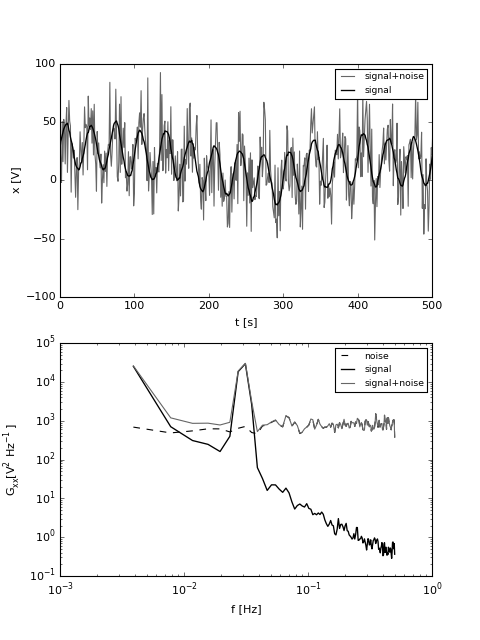Out:
<matplotlib.legend.Legend at 0x11011d290>

The most naive filter is the boxcar filter, or a moving average. For that, we choose a filter length that will keep the peak, but will remove most of the noise. For the example above, the peak is burried in the noise at $f=0.03\ \mathrm{Hz}$, so we might want a boxcar that is 25-s long, or for this 1-Hz data 25 data points long. This would put an ideal filter at $f=0.04\ \mathrm{Hz}$, or just above our peak. Lets see the effect of applying that: $$y_n = \sum_{k=0}^{24} \frac{1}{25}x_{n+k}$$

In :
y=0.*xn
# do the running average:
for n in range(0,N-1,1):
y[n]=sum(xn[n:(n+25)])/25.
py,f = mlab.psd(y,NFFT=nfft,Fs=1./dt)
def plotxyn(nosec=False):
fig,ax=plt.subplots(2,1,figsize=(6,8))
ax.plot(t,xn,color="0.4",label="signal+noise")
ax.plot(t,x,'k',linewidth=1.2,label="signal")
ax.plot(t,y,'r',linewidth=1.2,label="filtered s+n")
ax.set_xlim((0,500))
ax.legend(fontsize='small')
ax.set_xlabel('t [s]')
ax.set_ylabel('x [V]')
# spectrum
if nosec:
return
ax.loglog(f,pn,'k--',label="noise")
ax.loglog(f,px,'k',linewidth=1.2,label="signal")
ax.loglog(f,px+pn,color="0.4",label="signal+noise")
ax.loglog(f,py,'r',linewidth=1.2,label="filtered")
ax.set_xlabel('f [Hz]')
ax.set_ylabel(r'$G_{xx} \mathrm{[V^2 Hz^{-1}]}$')
ax.legend(fontsize='small')
ax.set_ylim((1.e-2,10.e5))
ax.legend(loc=3,fontsize='small')
return fig,ax
plotxyn()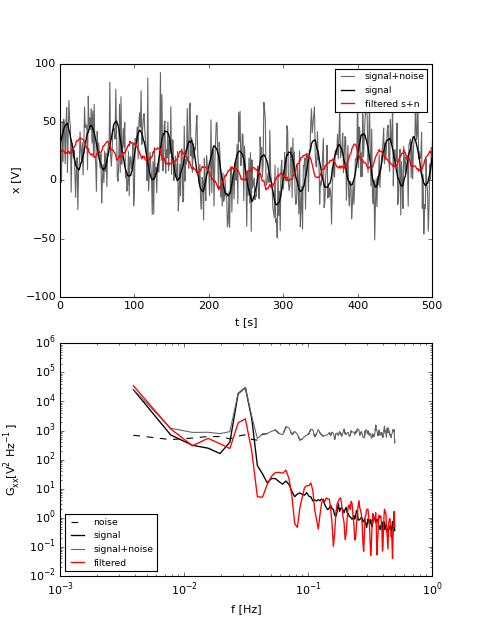Out:
(<matplotlib.figure.Figure at 0x10f32d450>,
array([<matplotlib.axes._subplots.AxesSubplot object at 0x1104a6b50>,
<matplotlib.axes._subplots.AxesSubplot object at 0x1107c0690>], dtype=object))

So, that was a reasonable guess. Certainly the low-frequencies are better resolved. But, it really attenuated the sine-wave. The sine wave also looks like its suffered a phase shift, which we may have expected from the fact that $y_1$ is the sum of $x_1$ to $x_{25}$.

We can emperically consider just the filter response by noting that $y=h*x$, so the Fourier transform is given by $Y(f)=H(f)X(f)$, and the filtering is just a linear system. Then emperically, $|H(f)|=\sqrt{G_{yy}/G_{xx}}$, and we get the phase of the filter from noting that:

$$X^*(f)Y(f) = H(f) X^*(f)X(f)$$

Or $angle(H(f)) = angle(G_{xy})$

In :
Gxy,f  = mlab.csd(xn,y,Fs=1./dt,NFFT=nfft)
def plotit():
fig,ax=plt.subplots(2,1,figsize=(5,6))

ax.loglog(f,np.sqrt(py/(px+pn)),'k')
ax.set_ylim((1.e-2,4))
ax.set_ylabel(r'$|H(f)|$')

ax.semilogx(f,np.angle(Gxy),'k')

ax.semilogx(f,np.mod(2*np.pi*f*25./2.,np.pi),'k--')
ax.set_ylabel('phase')
ax.set_xlabel('f [Hz]')
return fig,ax
plotit()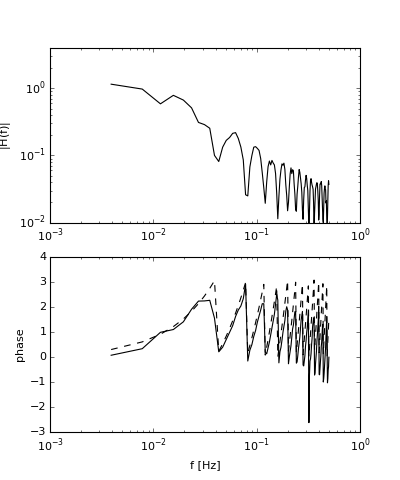Out:
(<matplotlib.figure.Figure at 0x10e549590>,
array([<matplotlib.axes._subplots.AxesSubplot object at 0x11259b610>,
<matplotlib.axes._subplots.AxesSubplot object at 0x112819d50>], dtype=object))

We can compute the frequency response of $H_m=H(f_m)$ where $f_m = \frac{m}{N}$ by taking the discrete Fourier transform of $h_k$:

\begin{align} H_m =& \frac{T}{N}\sum_{k=0}^{K-1} h_k \mathrm{e}^{\frac{-j2\pi km}{N}} \end{align}

, where $K$ is the length of the filter.

For the boxcar, $h_k=1/K$, so

\begin{align} H_m =& \frac{T}{KN}\sum_{k=0}^{K-1} \mathrm{e}^{\frac{-j2\pi km}{N}} \end{align}

This is just a geometric series, for which

$$\sum_{k=0}^{K-1} r^k = \frac{1-r^K}{1-r}$$

so

\begin{align} H_m =& \frac{T}{KN} \frac{1- \mathrm{e}^{\frac{-j2\pi Km}{N}}}{{1- \mathrm{e}^{\frac{-j2\pi m}{N}}}}\\ =& \frac{T}{KN}\mathrm{e}^{\frac{-j2\pi (K-1)m}{N}} \frac{\sin\left(\pi mK/N\right)}{\sin\left(\pi m/N\right)} \end{align}

This is called the Dirichlet Kernel, and is a fundamental function of time series analysis. It looks just like the sinc function for the continuous Fourier transform of a rectangle function discussed previously.

Applied to $K=25$ it looks like:

In :
m = np.array(range(1,N/2))
K=25.
H = 1./K*np.sin(np.pi*m*K/N)/np.sin(np.pi*m/N)

fm = m/dt/(1.*N)
# redo the last plot
fig,ax=plotit()
ax.loglog(fm,abs(H),'k--')
ax.set_ylim((1e-3,4.))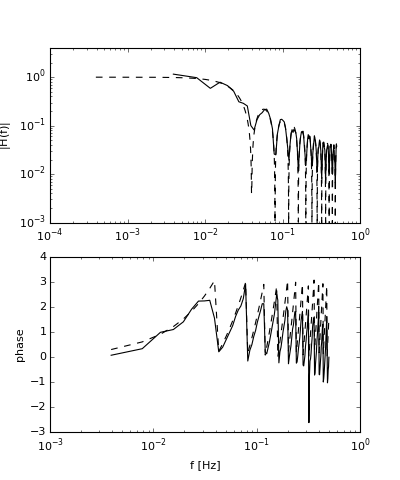Out:
(0.001, 4.0)

Note that the complex part of the filter response function $H_m$ leads to a linear phase offset as a function of frequency.

Here we see that the theoretical response is echoed in the filtered data response as we might expect.

This response has two problems:

1. There is significant "leakage" to high frequencies
2. There is a significant phase shift.



The phase shift is actually less of a problem than it appears. We could redefine our filter to be properly centered on the data, and the phase shift would go away. The poor high-frequency roll off is fixed the same way we fixed it when we fixed leakage in the Fourier transform: by applying a suitably tailored filter that the has better roll-off characteristics.

#### Carrying on with example¶

The passband is clearly lower than our hoped-for 0.04 Hz. In fact that is the first null of the filter. We also note a phase offset that looks very regular. In fact it can be shown that a phase offset of $\phi = \delta t (2\pi f)$ is expected, where $\delta t$ in this case is 25/2= 12.5 s. This phase offset can easily be removed by centering the filter on the data.

Also, a better filter for keeping the peak would be closer to 12 samples. We'll choose 13 samples to make a symmetric filter. While we are at it, lets center the filter.

In :
y=0.*xn
filtl=12 #+1
ind = np.array(range(-filtl/2,filtl/2+1))
print ind
for n in range(filtl/2,N-filtl/2,1):
y[n]=np.sum(xn[n-ind])/(1.*filtl+1)
py,f = mlab.psd(y,**args)
plotxyn()

[-6 -5 -4 -3 -2 -1  0  1  2  3  4  5  6]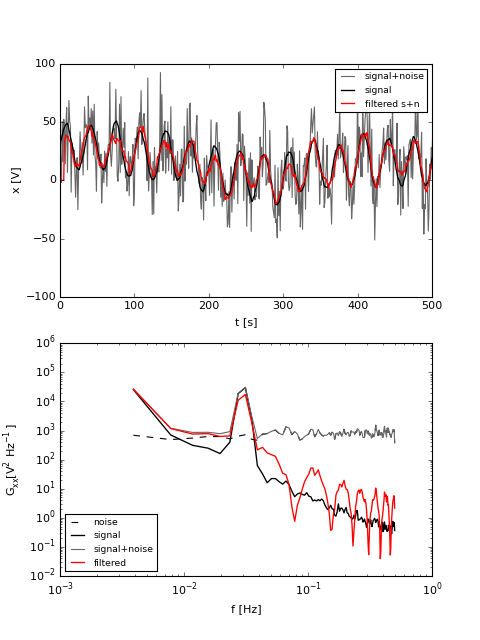Out:
(<matplotlib.figure.Figure at 0x1120bc790>,
array([<matplotlib.axes._subplots.AxesSubplot object at 0x113454a90>,
<matplotlib.axes._subplots.AxesSubplot object at 0x11349b590>], dtype=object))

Centering gets rid of the phase lag (as you might expect), and the better choice of frequency allows more of the sinusoid to come through, though there is still some attenuation.

## Simple filter in frequency space¶

So, the boxcar in time is non-ideal: it rolls off quite slowly in frequency space and doesn't squelch the high frequencies as well as we might like. How about another approach, filtering in frequency space? Here we simply calculate the Fourier transform of $x(t)$, remove the frequencies with $f>0.04 Hz$, and then do an inverse Fourier transform:

In :
X = np.fft.fft(xn)

N = np.shape(xn)
print dt
print nfft
ff = np.linspace(0,1./dt,N)
# Note that because the FFT is symetric we have to mask some of the high frequencies as well
X[(ff>0.04) & (ff<(1./dt-0.04))]=1.e-15
y = np.real(np.fft.ifft(X))+np.mean(xn)
py,fff = mlab.psd(y,**args)
xn0=xn*1.0
x0=x*1.0
plotxyn()

1.0
256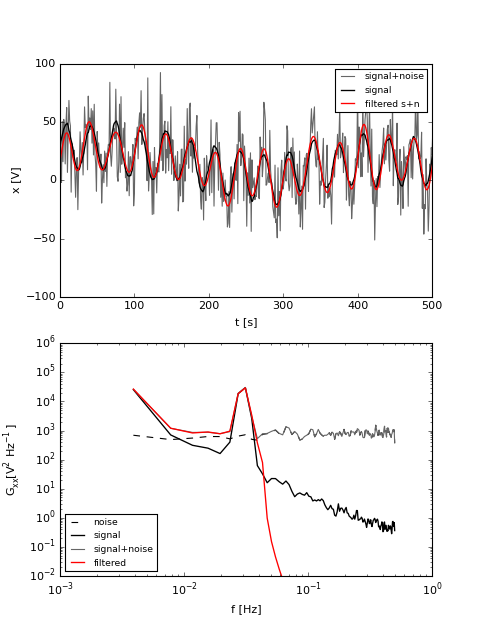Out:
(<matplotlib.figure.Figure at 0x112e20550>,
array([<matplotlib.axes._subplots.AxesSubplot object at 0x10e7a49d0>,
<matplotlib.axes._subplots.AxesSubplot object at 0x114074f90>], dtype=object))

So, this works pretty well for this time series, though we can see there are some parts of the signal that are not precisely followed. So why not filter exactly like this? For the same reason it doesn't work well to filter with a boxcar in the time domain. Consider a sharp step in our time series:

In :
xn=1.0*xn0
x = 1.0*x0
# put s sudden jump in
xn[300:-1]=xn[300:-1]-1000.
x[300:-1]=x[300:-1]-1000.
X = np.fft.fft(xn)
N = np.shape(xn)
print dt
print nfft
ff = np.linspace(0,1./dt,N)
# Note that because the FFT is symetric we have to mask some of the high frequencies as well
X[(ff>0.04) & (ff<(1./dt-0.04))]=1.e-15
# There is a strange bug in psd that makes adding this little bit of noise necessary.
y = np.real(np.fft.ifft(X))+1.e-17*np.random.randn(N)

py,fff = mlab.psd(y,**args)

plotxyn(nosec=True)

1.0
256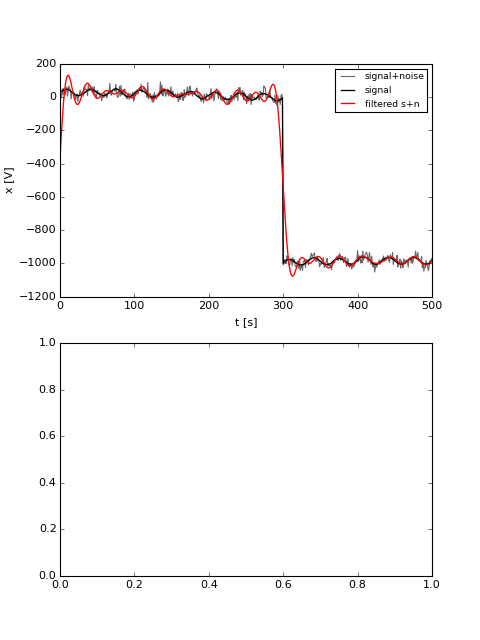Note the sharp overshoot at the step. This is "ringing" or "ripple" is due to the overly sharp frequency filter. Again, the solution is to window in frequency space (see exercise).

## Filter design: Finite amplitude response (FIR)¶

If the Boxcar is too crude for our needs, then we need to design something more sophisticated. The simplest idea is to consider a boxcar in frequency space: $H_m = 1$, for $|m| \lt (M-1)/2$, $H_m=0, |m| \gt (M-1)/2$. Here, $(M-1)/2 = f_c T$ is the integer representing $f_M>=f_c$, our design cutoff. This gives us a Dirichlet Kernel in the time domain:

$$h_k = \frac{1}{N}\frac{\sin(\pi k M/N)}{\sin(\pi k/N)}$$

Note that here the phase information in the kernel has been ignored, except for the plus or minus due to the sin. Thus we already can predict a problem with this style of filter: that it will not have the phase information correct.

Choosing some numbers, imagine the time series is $N=161$ data points long, each sampled $\delta t=1 \mathrm{s}$ apart and we want to filter at 0.1 Hz. Then $M = 2 f_c T +1=2 f_c/f_S N=31$. Plotting it in time and frequency:

In :
N=161
fs=1.
fc = 0.1/fs

M = fc*2*N+1
k = np.array(range(-N/2+1,N/2))
m= k*1.
H = 0.*k+1.
H[(np.abs(m)>(M)/2)]=0.
H1=H
## Note the use off fftshift.  Usually fft and ifft assume that k=0
# is the first element in the vector, and k=N is the last.
# fftshift puts k=-N/2 as the first, and k=+N/2-1 as the last.
h1 = np.abs(np.fft.fftshift(np.fft.ifft(np.fft.fftshift(H))))
# trick!  the Dirichlet kernal has singularities at integer values of k,
# so we add very small numbers to k get rid of these:
h2 = (1./N)*np.sin(np.pi*(k+0.000001)*M/N)/np.sin(np.pi*(k+0.000001)/N)
H2 = np.fft.fftshift(np.fft.fft(h2))
fig,ax=plt.subplots(2,1,figsize=(5,6))
ax.plot(m,H,'dk',markersize=4)
ax.plot(m,H,'k')

ax.plot(m,abs(H2),'r')
ax.set_ylim((0,1.1))
ax.set_ylabel(r'$H_m$')
ax.set_xlabel(r'frequency: $m$');ax.set_title('Frequency Response',loc='left')
#xlim((-20,20))
ax.plot(k,h2,'dk',markersize=4)
ax.plot(k,h2,'k',label='Dirchlet kernel')
ax.plot(k,h1,'r',label='Inverse fft of step function')
ax.set_title('Filter in time domain',loc='left')
plt.ylabel(r'$h_k$')
plt.xlabel(r'time: $k$')
plt.ylim([-0.04,0.23])
plt.tight_layout()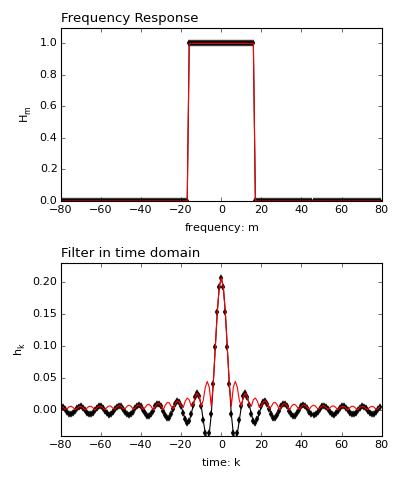Note that both the frequency and the time response have length $N=161$. (Also note the use of fftshift to return vectors centered on zero.)

The ideal filter in the frequency domain (index $m$) is the Dirichlet kernel in the space domain (index $k$). However, we cannot filter with this raw $h_k$ because it has length $N$, and that is how long our time series is! So we have to truncate $h_k$, and that of course leads to imperfections in the frequency domain.

Consider the case where the length of the time series is $N=161$, and lets look at the effect of truncating the filter $h_k$ to have 101, 31, 19, 11 and 5 elements:

In :
N=161

M = fc*2*N+1
h2 = (1./N)*np.sin(np.pi*(k+0.000001)*M/N)/np.sin(np.pi*(k+0.000001)/N)
k = np.array(range(-N/2+1,N/2))

H1 = h2*0+1.
H1[abs(k)>(M-1)/2]=0.
fig,ax=plt.subplots(2,1,figsize=(5,6))
ax.plot(k,H1,'k--')
for K in [101,31, 19, 11, 5]:
h = h2
h[abs(k)>(K-1)/2]=0
H = N*np.fft.fftshift(np.fft.ifft(h))

ax.plot(k,abs(H),label='%d' % K)
ax.plot(k,h)
ax.legend()
ax.set_ylabel(r'$h_k$');ax.set_xlabel(r'time: $k$');ax.set_title('Frequency Response',loc='left')
ax.set_ylabel(r'$H_m$');ax.set_xlabel(r'frequency: $m$');ax.set_title('Dirichlet Kernel Filter in time domain',loc='left')

plt.tight_layout()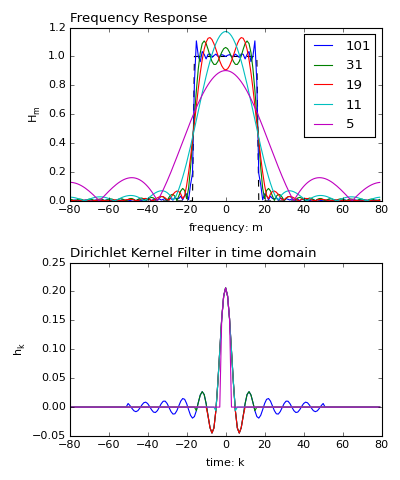As the truncated Dirichlet kernel gets smaller, the roll-off gets less steep and the passband ripple size gets larger. Even for a kernel with 101 entries, there is a substantial ripple at the edges of the passband, an effect that is often un-desireable. There is usually a trade-off in designing FIR filters between the amplitude of the passband ripple and the sharpness of the roll-off.

One approach to reducing the ripple is to window the truncated dirichlet kernel. If $h_k^K$ is the Dirichlet kernel of length $K$, then we choose the Hanning window of length $K$ to smooth out the passband ripple and form a new filter: $h'_k=h_k^K w^K$:

In :
h2 = (1./N)*np.sin(np.pi*(k+0.000001)*M/N)/np.sin(np.pi*(k+0.000001)/N)
H1 = h2*0+1.
H1[abs(k)>(M-1)/2]=0.
fig,ax=plt.subplots(2,1,figsize=(5,6))
ax.plot(k,H1,'k--')
for K in [101,31, 19, 11, 5]:
h = 1.0*h2
h[abs(k)>(K-1)/2]=0
w = 0.*h
w[abs(k)<=(K-1)/2]=np.hanning(K)
H = N*np.fft.fftshift(np.fft.ifft(h*w))
ax.plot(k,abs(H),label='%d' % K)
ax.set_xlabel('m ')
ax.set_ylabel('$H_m$')
ax.plot(k,h*w)

ax.set_xlabel('k')
ax.set_ylabel('$h_k$')
K=19
h = 1.0*h2
h[abs(k)>9]=0
H = N*np.fft.fftshift(np.fft.ifft(h))
ax.plot(k,abs(H),'r--',label='19 - no wind')
ax.legend(fontsize='small')
ax.plot(k,h,'r--')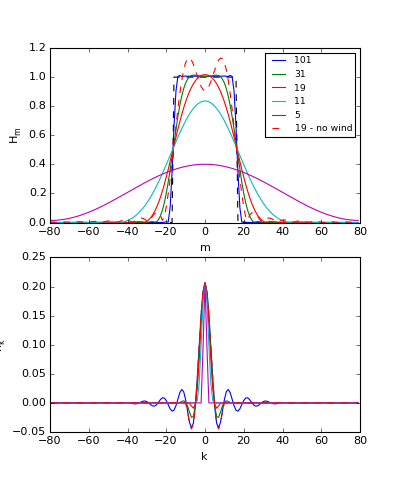Out:
[<matplotlib.lines.Line2D at 0x119325190>]

Comparing the windowed version for $K=19$ (red solid line) to the un-windowed filter (red dashed line), we see that the roll-off suffers, but the ripple is greatly reduced. There is no free lunch in filter design!

### Example Truncated Dirichlet Kernel Filter¶

In :
# redoing our example:
N=256*10
dt=1.
t=np.arange(0,N*dt,dt)
x = np.cumsum(np.random.randn(N)) # red noise
f0=0.03 # Hz
x = x+ 20.*np.sin(f0*2*np.pi*t) # + sine wave
x=x-np.mean(x)
noise = 20.*np.random.randn(N)
xn = x+noise
# remove the mean!!
xn = xn-np.mean(xn)
#
# Calculating the truncated Dirichlet Kernel to filter at 0.05 Hz lowpass:
k = np.array(range(-N/2+1,N/2))
fc = 0.05
M = fc*2*N+1
h2 = (1./N)*np.sin(np.pi*(k+0.000001)*M/N)/np.sin(np.pi*(k+0.000001)/N)
fig,ax=plt.subplots(1,1)
ax.plot(k,h2)
ax.set_xlabel('k')
ax.set_ylabel('$h_k$')
ax.set_xlim([-100,300])
ax.set_title('%f Hz low pass'%fc)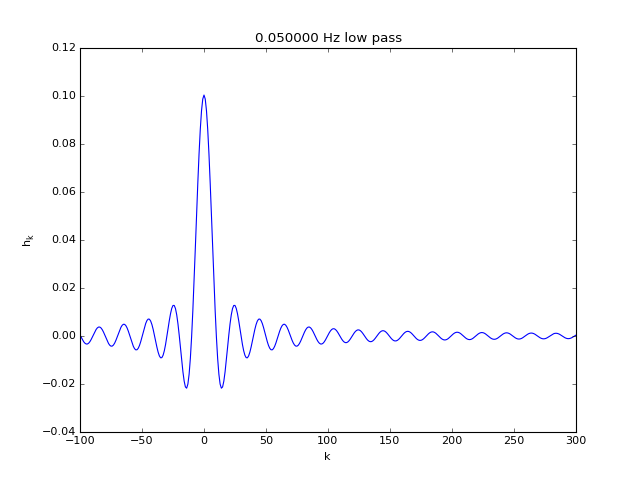Out:
<matplotlib.text.Text at 0x119e614d0>

So, to get a 0.05 Hz low-pass we will need a filter that is at least 40 points wide; lets use 120:

In :
h = h2*1.
# how long is the filter:
filtlen=60
h = h[abs(k)<filtlen+1]
fig,ax=plt.subplots(1,1)
ax.plot(k,h2)
ax.set_xlabel('k')
ax.set_ylabel('$h_k$')
ax.set_xlim([-100,300])
ax.set_title('%f Hz low pass'%fc)
ax.plot(k[abs(k)<filtlen+1],h,'r')